# Convolution Theorem

## Convolution Theorem:

The convolution theorem of Laplace transform states that, let f1 (t) and f2 (t) are the Laplace transformable functions and F1 (s), F2 (s) are the Laplace transforms of f1 (t) and f2 (t) respectively. Then the product of F1 (s) and F2 (s) is the Laplace transform of f(t) which is obtained from the convolution of f1 (t) and f2 (t). The convolution of f1 (t) and f2 (t) is denoted as f1 (t) * f2 (t) and is obtained by the equation,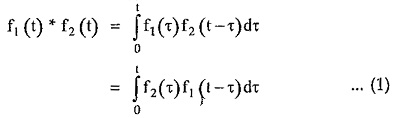where τ is the dummy variable.where f1 (t) * f2 (t) indicates convolution of f1 (t) and f2 (t).

Proof:

Let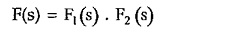Where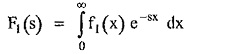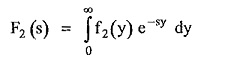x and y are dummy variables.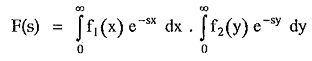As x and y are independent variables we can write,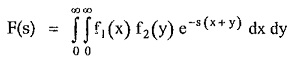Consider the new variables t and τ such that,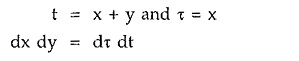Let us consider limits of integration interms of t and τThe smallest value of y is zero hence t ≥ τ.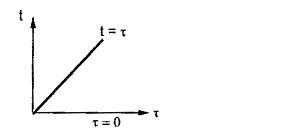The equation t = τ is straight line in t – τ plane. So to integrate area between the line t = τ and τ = 0, we get the limits for t as 0 →∞ while limits of τ as 0 to t.

Now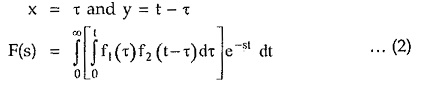From the definition of Laplace transform the equation (2) represents Laplace transform of integral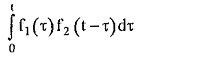which is called convolution integral. So in the equation (1),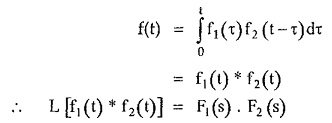Thus explained.

Updated: October 11, 2019 — 11:30 pm